# Volume of Cylinders, Cones, and Spheres Lesson Plan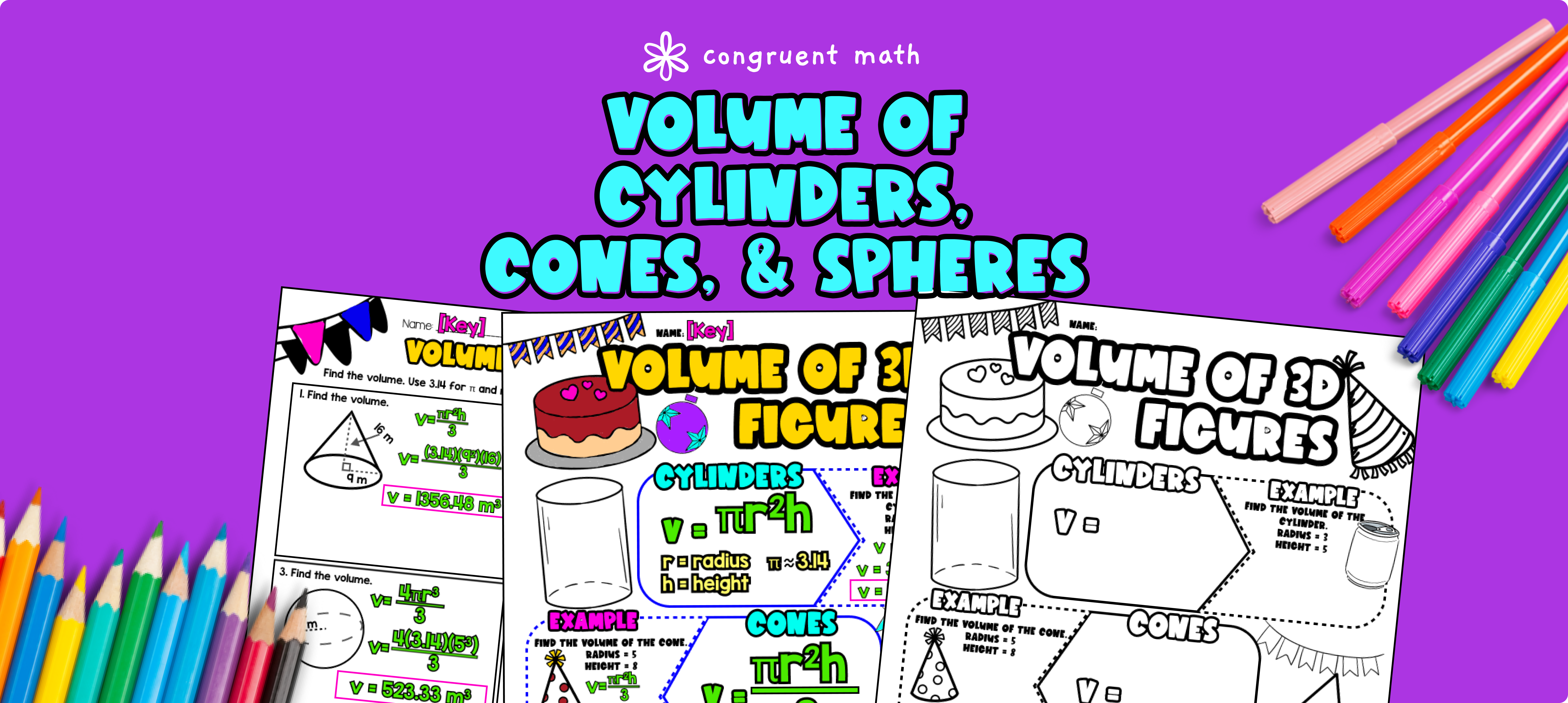Pin This

## Overview

Ever wondered how to teach volume calculations of cylinders, cones and spheres in an engaging way to your 8th grade students? In this lesson plan, students will learn about volume calculations and their real-life applications.

Through artistic, interactive guided notes, check for understanding, maze, doodle & color by number activities, and real-life application of paint in a can, students will gain a comprehensive understanding of volume calculations.

The lesson culminates with a real life example that explores how the volume calculation is used in measuring paint in a can.

## Get the Lesson Materials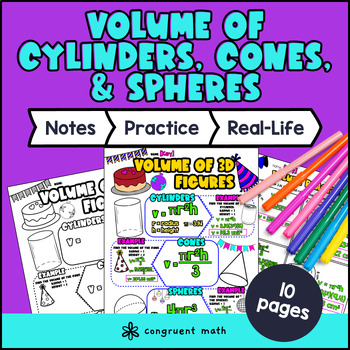\$4.25

## Learning Objectives

After this lesson, students will be able to:

• Know the formulas for the volume of cylinders, cones, and spheres

• Calculate the volume of cylinders, cones, and spheres

• Apply the concept of volume to real-life situations, such as measuring the volume of paint in a can.

## Prerequisites

Before this lesson, students should be familiar with:

• Basic multiplication and division skills

• How to evaluate exponents (powers of 2 and 3)

• Basic understanding of fractions and decimals (optional, but helpful)

• Volume

• Cubic Units

• Cylinder

• Cone

• Sphere

• Exponents

## Procedure

### Introduction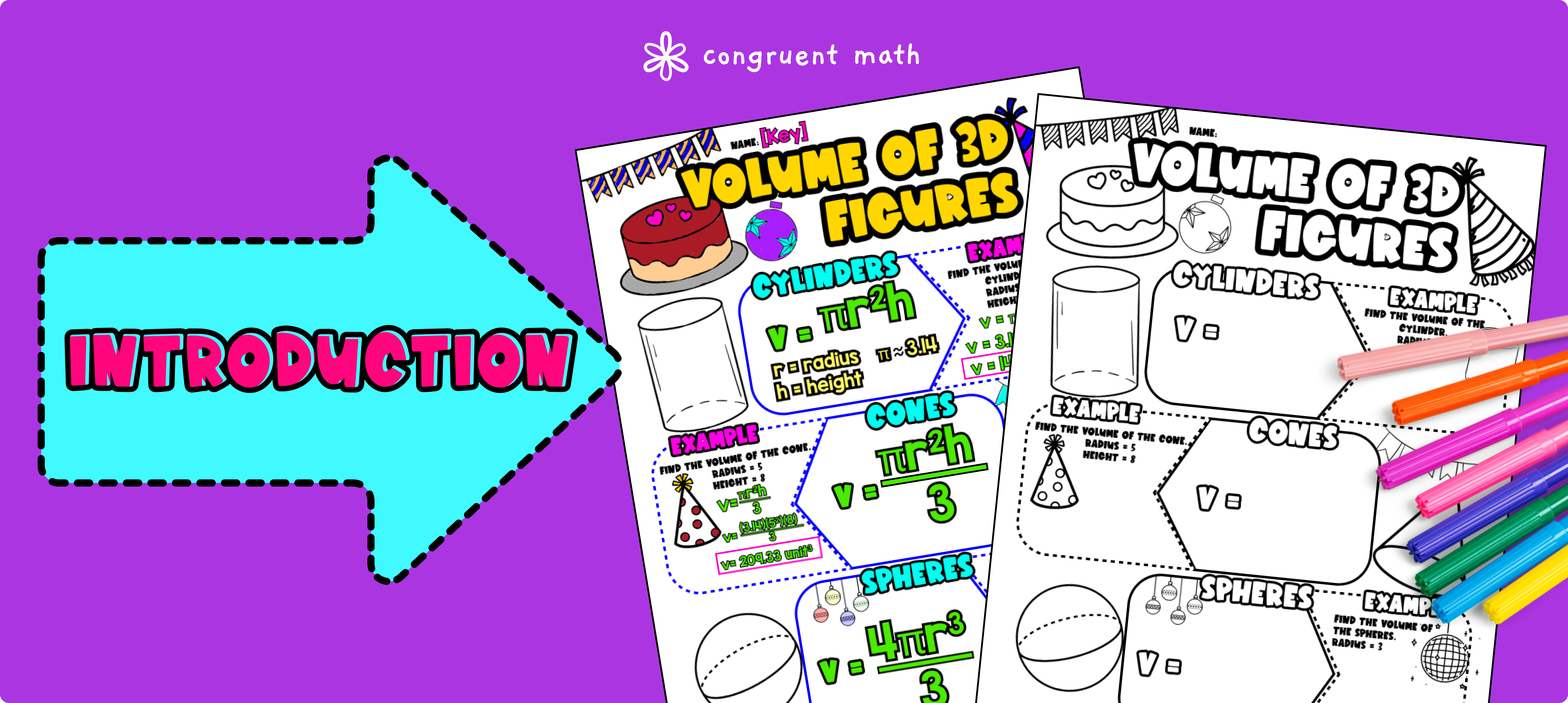Pin This

As a hook, ask students why it is important to be able to calculate the volume of objects like cylinders, cones, and spheres. Refer to the last page of the guided notes as well as the FAQs below for ideas.

Use the guided notes to introduce the concept of finding volume of cylinders, cones, and spheres. Walk through the key points of the topic of the guided notes to teach. Refer to the FAQ below for a walk through on this, as well as ideas on how to respond to common student questions.

Have students walk through the “Volume Practice” section. Call on students to talk through their answers, potentially on the whiteboard or projector. Based on student responses, reteach concepts that students need extra help with.

Based on student responses, reteach concepts that students need extra help with. If your class has a wide range of proficiency levels, you can pull out students for reteaching, and have more advanced students begin work on the practice exercises.

### Practice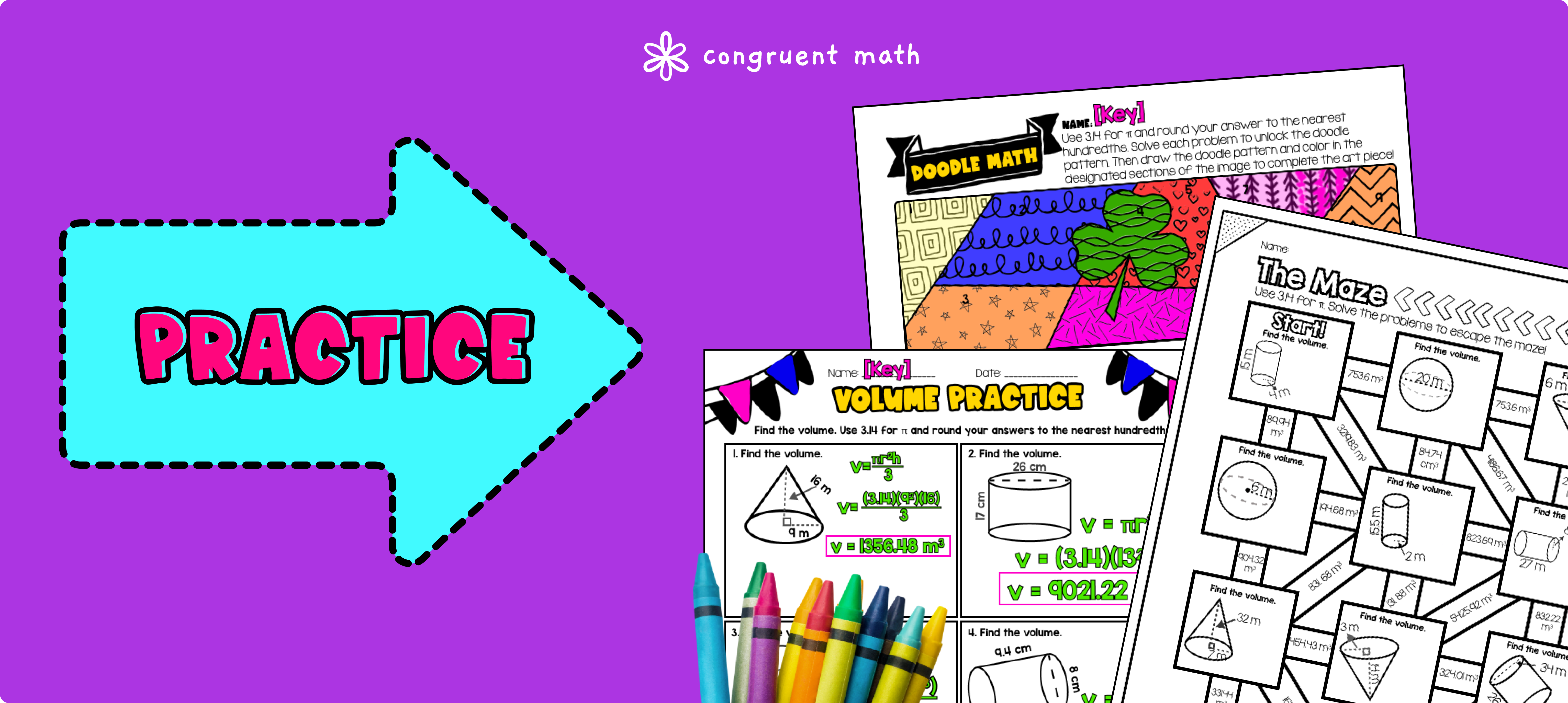Pin This

Have students practice finding the volume of cylinders, cones, and spheres using the Doodle Math activity. Walk around to answer student questions.

Fast finishers can dive into the maze for extra practice. You can assign it as homework for the remainder of the class.

### Real-Life Application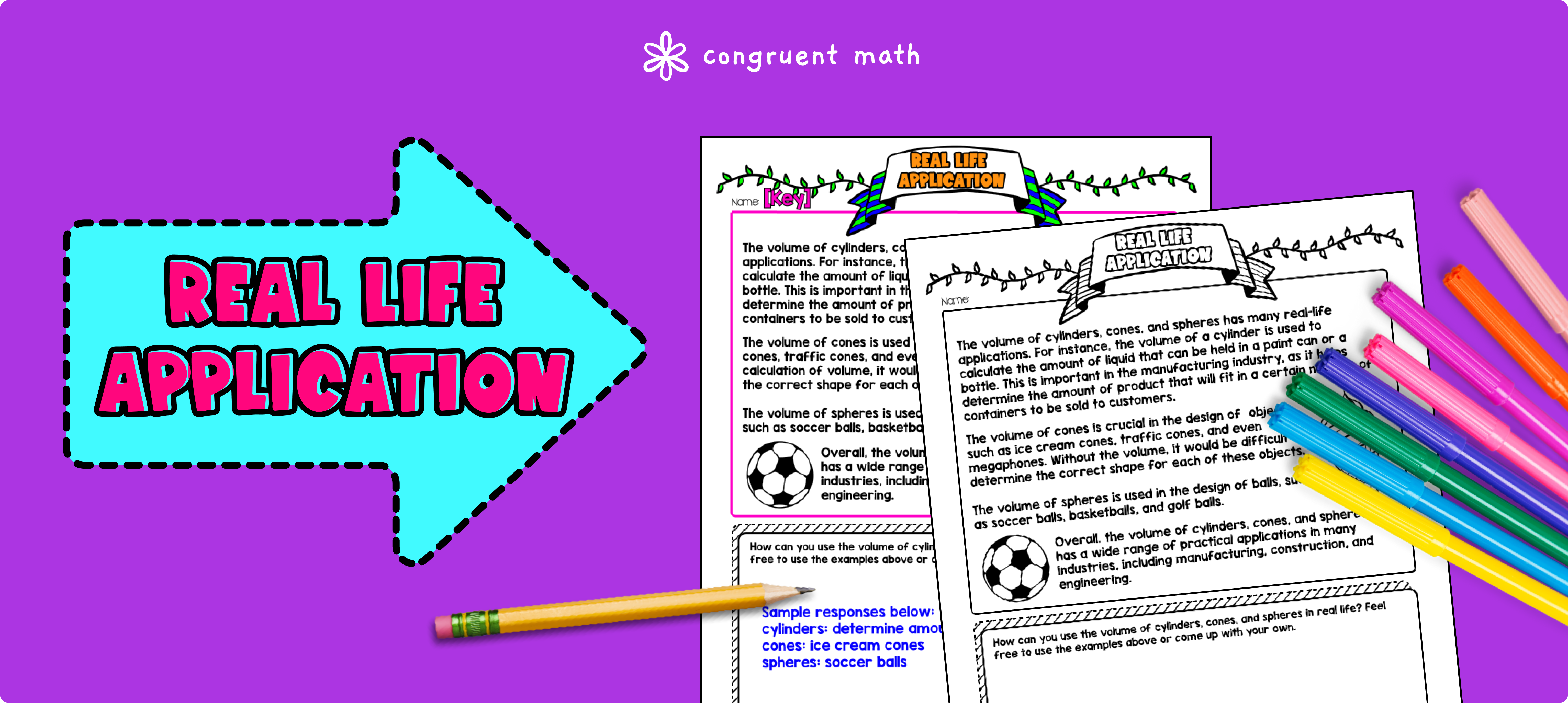Pin This

Bring the class back together, and introduce the concept of measuring the volume of paint in a can. Explain how understanding the volume of the can and the amount of paint inside can help determine how much paint is needed for a project, and how it can save time and money by avoiding over-purchasing paint.

Refer to the FAQs below for more ideas on how to teach this real-life application.

## Extensions

If you’re looking for digital practice for volume calculations of cylinders, cones, and spheres, try the Pixel Art activities in Google Sheets. Every answer is automatically checked, and correct answers unlock parts of a mystery picture. It’s incredibly fun and a powerful tool for differentiation.